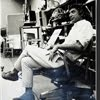## Eugene Ray SDSU 1970'sEugene Ray 1970's. photo credit: Tom Davis

## Monday, October 1, 2012

#1
+INVENTION+
>blueprint background for color<
>rendering with prismacolor<
^^^^^^^^^^^^^^^

>G R A T I T U D E+
>again to david fobes for his great<
>effort to aid my ability to show<
+MY WORK TO THE WORLD+
^^^^^^^^^^^^^^^
+EUGENE RAY.ARCHITECT.COM+
(>eugenerayarchitect.blogspot.com<)
^^^^^^^^^^^^^^^^^^^
+HISTORICAL MOMENT+
>and find the new web/blog spot<
>dave has arranged for me<
^^^^^^^^^^^^^^

+GRAND VISION+
>although my art & architecture<
>archive is destined to go to<
+S.D.S.U+
>(california work)<
>and<
+T.H.N.O.C.+
>(louisiana work)<
^^^^^^^^
>i have arranged to have dave act<
>as my living agent when i go to<
+ANOTHER DIMENSION+
^^^^^^^^^^^^^
>thus an expanded generation of<
>publication and exhibition can<
+CARRY THE WORK+
^^^^^^^^^^^^^^^

+I HAVE A VAST ARCHIVE OF+
>plus notebooks<
>letters etc<
+METAPHYSICAL GRAPHICS+
>architectural<
^^^^^^^

+PERSPECTIVE+
>as many of you who have known<
>me for a very long time know<
+MIDNIGHT MUSING+
>has attempted to show the<
>historical roots of my<
+O E U V R E+
^^^^^^
>france, switzerland, italy, spain<
>england and new orleans<
+ALL PLAY VITAL ROLES+
^^^^^^^^^^^

>mexico, haiti and french west indies<
>are also very inportant as is distant<
+J A P A N+
(>i have not written recently about<
^^^^^^^^^^^^^^
+HAIKU POETRY+
>and<
+RYOANJI & ISSE+
+BASHO & HOKUSAI+
>and<
^^^^^^^^^
>but alumni know this was vital<
>to my work and teaching<)
^^^^^^^^^^^^^^
+REMEMBER THE KOTO SOUND+
>moonlight nights<
+IN THE SANSOUCI FOREST+
^^^^^^^^^^^^^^^
>these topics shall be in future<
+TRANSMISSIONS+
>as shall my great surreal photo<
>friend from new orleans<
^^^^^^^^^^^^^
+CLARENCE JOHN LAUGHLIN+
^^^^^^^^^^^

+THE HISTORIC NEW ORLEANS+
>prints/negs/papers<
+COLLECTION HAS HIS WORK+
^^^^^^^^^^^^^^^
>thanks to friend john h. lawrence<
>who we shall see in new orleans<
>at the end of this month<
^^^^^^^^^^^^^

+TONIGHTS PHOTOS+
>five images dave photographed<
+V A R I E T Y+
>of my architecture in california<
^^^^^^^^^^^^^^^^^

#1)&#4)   +INVENTION+
>blueprint background for color<
>rendering with prismacolor<
^^^^^^^^^^^^^^^
>two renderings of my designs with<
>prismacolor on blueline print<
>using a lithographic neg<
^^^^^^^^^^^^^^

#1)&#5)  +VERSATILITY+
>the first a "low tech" solution<
+BIOMORPHIC+
>the second a "high tech"<
+SOLAR+
>under openable roof domes<
^^^^^^^^^^^^^^^^

#3)          +REALIZATION+
>although my major teaching thrust<
>was for cutting edge research of<
+PROTOTYPES+
>to improve the art of advanced<
+ARCHITECTURE+
^^^^^^^^
>i answered all invitations to build<
+"YOGURT ENCOUNTERS"+
>for larry uruttia (mfa/ed) was<
>one of those invitations<
^^^^^^^^^^^^^^

+NOTE : MARIANNE & I GO TO+
>annual gathering of<
>her classmates<
+SANTA BARBARA TUESDAY+
^^^^^^^^^^^^^^^
>we shall be gone for<
+FOUR DAYS+
^^^^^^
gene ray, lajolla, california

>eugene ray, mfa, architect<
>professor emeritus, sdsu<
^^^^^^^^^^^^^^